`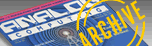A.N.A.L.O.G. ISSUE 62 / JULY 1988 / PAGE 29`

 48k disk or cassette The Magic Of Tesselations -Part II by Allan Moose and Marian Lorenz The theme of distorting a basic tile shape as it is drawn in successive rows forms the basic idea behind many of M.C. Escher's drawings, A tesselation or tiling is the complete covering of a flat surface by one or more figures in a pattern, with no overlapping of the figures and no open spaces. As we discussed last month, tesselations are commmonly found in linoleum patterns, parquet floors or fabrics.     All of the tilings we discussed, and many of the tilings found around one's home, share the property of being periodic. A tesselation is periodic if you can shift the drawing without rotation or reflection to a new position where all outlines again fit exactly. While there are an infinite number of shapes that will tesselate periodically, periodic tilings by no means exhaust all of the possible ways to cover a plane surface. In this article we will present two programs that illustrate more intricate tilings. The first draws a non-periodic tiling that has rotational symmetry. The second covers the screen with tiles that are gradually deformed as each successive row is drawn on the screen.     One of the charming things about the study of tilings is that it bridges the gap between art and science. Mathematicians have related tilings to group theory, which is the abstract study of symmetry. Physicists study tesselations to gain insights into the formation of crystals, and, of course, the famous artist M. C. Escher made frequent use of tilings in his work. In developing our programs we drew upon ideas from all of these fields. In particular, we have made use of the ideas of translation and rotation of coordinates as a way of writing short programs that you may easily modify.     In order to illustrate tesselations with rotational symmetry, the basic tile used is a diamond which is based on a 30-60-degree right triangle.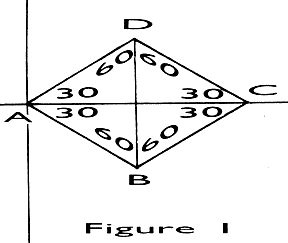Previously, we introduced the use of a "local coordinate system" (LCS). The idea behind a LCS is that if you want to repeatedly draw the same figure on the CRT screen, the most efficient way to do it is to represent the coordinates o£ the vertices of the figure (points A,B,C,D in Figure 1) in terms of a hypothetical coordinate system. Then to draw the figure on the screen all you do is position the origin of the LCS where you want it and draw. In this way, the same subroutine can draw all the tiles you need.     In the LCS, the coordinates of the vertices of Figure 1 are: A = 0, 0 B = 17.32, -10 C = 34.64, 0 D = 17.32, 10 To make a tesselation with rotational symmetry, we want to draw this diamond in a circular pattern so that the first row will look like: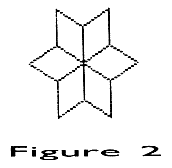The next circular row must fit around this design. The algorithm for generating the pattern can be simply stated as:     READ, ROTATE, TRANSLATE.     That is, for each diamond, no matter where it is, the program first reads the data numbers, then rotates the figure into the proper position, and then translates the vertex of the diamond to the proper location.     With this background, you should be able to follow the program of Listing 1. This program creates three rows of diamonds (Figure 3). You may easily modify it to add a fourth. Be sure to include a clipping routine to avoid the dreaded "cursor out of range" error!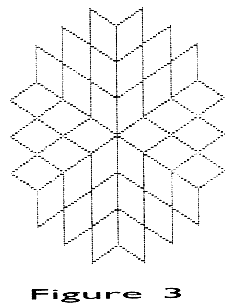Of course, you will want to experiment with more than just diamond tiles. An excellent place to start is with triangle tilings. The reason is that each tile can be changed by distorting the legs. For example, change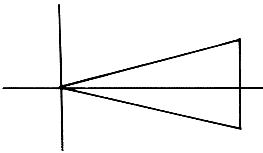to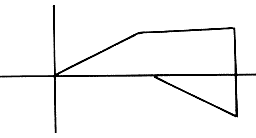by nending each leg from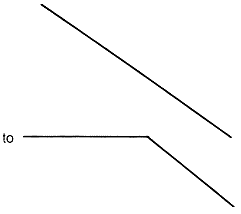Actually you can be more imaginative than this because a triangle can be distorted in an infinite number of ways to yield a figure capable of being used in a rotational tesselation.     The theme of distorting a basic tile shape as it is drawn in successive rows forms the basic idea behind many of M. C. Escher's drawings. For example, birds might gradually lose their shapes and become checkerboarded fields of hay or even metamorphose completely into fish as in "Sky and Water 1." Our second program illustrates this gradual metamorphosis of one shape into another. In addition to being indebted to M. C. Escher for inspiration, we also must credit Douglas Hofstadter who, several years ago, devoted a column of "Metamagical Themas" in Scientific American to "Parquet Deformations."     The basic idea is that simple geometric shapes which can tile the plane are slowly deformed as they move across or down the plane. Deformations may be created with a number of simple techniques such as: 1. Lengthening or shortening a line. 2. Introducing a "hinge" into a line segment so that it can flex. 3. Rotating a line or a group of lines that form a natural sub-unit. 4. Introducing a small "bump" or tooth into a line segment. By using one of these techniques and allowing it to continue long enough, such deformations can have unexpected results; one outcome being that tiles at the end of the work bear little or no resemblance to those at the beginning.     In order to keep our program simple, we restricted it to drawing a diamond and flexing the sides of the tile "in" or "out" to deform it. There are, of course, many other methods of deforming tiles, just as there are many other shapes that lend themselves to deformation. Here is a chance to exercise your creativity by building upon the ideas in this program.     It is evident that drawing a tesselation in which the shape of the tile is changed before each row is drawn is more involved than simply drawing the same tile many times. We have approached this problem by introducing what at first Physicists study tesselations to gain insights into the formation of crystals, glance may seem like an unnecessary complication. First, we note that many shapes which can tile a surface have some sort of rotational symmetry. This means that if you rotate the shape around its center through some fraction of a circle (1/2, 1/3, 1/6, etc.) you get back the original shape. If this is the case, and it certainly is with the diamond, then we need only specify part of the shape, say two sides, and let the computer rotate that part as often as necessary to close the shape. You should visualize this rotation as taking place in the LCS. The rotation routine necessary to do this is the same as the rotation subroutine in Listing 1, lines 140 and 150. If we must rotate the part of the shape N times to produce a closed figure, then the angles through which we must rotate it are multiples o£ 360/N. Having constructed the tile in a LCS, it may be easily translated to the proper positions and plotted on the screen.     Introducing the extra step of putting a tile together by rotation gives us a way to deform the tiles. Conceptually, deforming a tile is rather simple. Just take each side of the tile in turn, keeping the end points stationary, move the midpoint alternately in toward the center or out away from the center one unit at a time. The problem is in determining where to move the midpoint to. That is, given the coordinates of the end points, what are the coordinates of the point one, two, or three units closer to, or farther from the tile's center than the line's midpoint? If the line happens to be horizontal or vertical, then there is no problem. For example, a horizontal line's midpoint has the same ycoordinate as its end points. It has an x-coordinate equal to the average of the x-coordinates of the ends. Moving the midpoint toward or away from the center is a matter of moving it up or down. That is, the x-coordinate stays the same and the y-coordinate increases or decreases by one. If the line is diagonal we can still find the midpoint easily enough. However, moving it is the hard part, as the distance and direction of movement depend entirely on the inclination of the line segment.     It would be much easier for the purposes of this exercise if we could make each side horizontal long enough to deform it and then put it back where it belongs. Fortunately, we already have the tools available to do just that-local coordinates and rotation. In mathematical terms we want to: • Translate each line segment to the origin.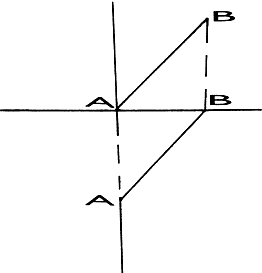• Rotate it onto positive x-axis,• Deform it as explained above.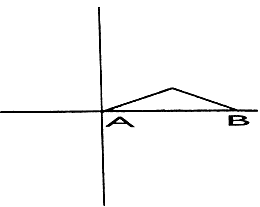• Rotate and translate it back into position.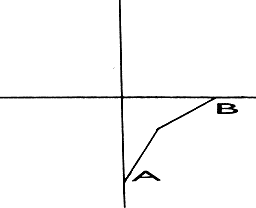For us the procedure is as follows: 1. Take each of the defined sides in turn. Pick one end point and call it X1, Y1. Call the other end X2, Y2. 2. If we shift the origin of the LCS to the point at X1, Y1 then the other point will have the coordinates (X2-X1), (Y2-Y1). 3. Find the inclination of the line or the angle theta which it makes with the xaxis. If the line is vertical, then THETA = 90. If the line slopes towards the right (x2 > x1), then THETA= ARCTAN ((Y2-Y1)/(X2-X1)). If the line slopes towards the left (X2XMAX THEN     XMAX=ABS(ARRAY1(VERTEX,1)) II 270 IF ABS(ARRAY1(VERTEX,2))>YMAX THEN     YMAX=ABS(ARRAY1(VERTEX,2)) AH 280 NEXT VERTEX BJ 290 HEIGHT=2*YMAX:WIDTH=2*XMAX SI 300 MAXCOLS=INT(320/WIDTH)-1 UJ 310 MAXROWS=INT(192/HEIGHT)-1 SR 320 INITIALX=XMAX+5:INITIALY=YMAX+5 QU 330 REM YF 340 REM **** PLOT THE FIRST ROW OF TIL    ES **** QY 350 REM HD 360 FOR COL=1 TO MAXCOLS UP 370 FOR THETA=0 TO 360 STEP THETAINC IE 380 FOR VERTEX=1 TO NUMVERTS ZU 390 X=ARRAY1(VERTEX,1):Y=ARRAY1(VERTEX    ,2) VO 400 GOSUB 80 LV 410 SCRNX=INITIALX+(COL-1)*WIDTH+XPRIM    E RM 420 SCRNY=INITIALY-YPRIME KH 430 IF VERTEX=1 THEN PLOT SCRNX,SCRNY:    GOTO 450 PL 440 DRAWTO SCRNX,SCRNY AD 450 NEXT VERTEX TU 460 NEXT THETA UN 470 NEXT COL RF 480 REM LY 490 REM **** DRAW SUCCEEDING ROWS OF T    ILES **** QQ 500 REM NA 510 FOR ROW=2 TO MAXROWS TQ 520 YOFFSET=YOFFSET+1 UU 530 GOSUB 730 HB 540 FOR COL=1 TO MAXCOLS UN 550 FOR THETA=0 TO 360 STEP THETAINC SP 560 FOR VERTEX=1 TO NUMVERTS*2-1 BJ 570 X=ARRAY2(VERTEX,1):Y=ARRAY2(VERTEX    ,2) WF 580 GOSUB 80 MM 590 SCRNX=INITIALX+(COL-1)*WIDTH+XPRIM    E EQ 600 SCRNY=INITIALY+(ROW-1)*HEIGHT-YPRI    ME PI 610 IF VERTEX=1 THEN PLOT SCRNX,SCRNY:    GOTO 660 YA 620 REM CLIPPING ROUTINE KP 630 IF SCRNX<0 OR SCRNX>319 THEN GOTO    940 JU 640 IF SCRNY<0 OR SCRNY>191 THEN GOTO    940 PP 650 DRAWTO SCRNX,SCRNY AH 660 NEXT VERTEX TY 670 NEXT THETA UR 680 NEXT COL FP 690 NEXT ROW QG 700 GOTO 940 QU 710 REM GL 720 REM **** SUBROUTINE TO CREATE AN A    RRAY OF DEFORMED TILES **** QY 730 REM HQ 740 FOR I=1 TO NUMVERTS-1 TP 750 X1=ARRAY1(I,1):ARRAY2(2*I-1,1)=ARR    AY1(I,1) MP 760 X2=ARRAY1(I+l,1) XP 770 Y1=ARRAY1(I,2):ARRAY2(2*I-1,2)=ARR    AY1(I,2) NR 780 Y2=ARRAY1(I+1,2) CH 790 X=X2-X1:Y=Y2-Y1 UE 800 IF X=0 THEN THETA=(-1)*SGN(Y)*98:G    OTO 850 QO 810 THETA=ATN(Y/X) OA 828 IF X<0 THEN THETA=180+THETA FM 830 THETA=-THETA:REM ROTATE TOWARD X-A    XIS WA 840 GOSUB 80 XL 850 X=XPRIME/2:Y=YOFFSET*(-1)^(I+1) LX 860 THETA=-THETA:REM ROTATE BACK INTO    POSITION WG 870 GOSUB 80 FD 880 ARRAY2(2*I,1)=XPRIME+X1:ARRAY2(2*I    ,2)=YPRIME+Y1 GO 890 NEXT I IV 900 REM **** COMPLETE ARRAY2 **** SU 910 FOR J=1 TO 2:ARRAY2(2*NUMVERTS-1,J    )=ARRAY1(NUMVERTS,J):NEXT J ZJ 920 RETURN MH 930 REM **** SCREEN DUMP **** UY 940 GOSUB 1080 MW 950 DIM GRAF\$(200) ZZ 960 GRAF\$(1)=CHR\$(0):GRAF\$(200)=CHR\$(0    ):GRAF\$(2)=GRAF\$ ML 970 LPRINT CHR\$(27);CHR\$(65);CHR\$(8) HH 980 SCRNMEM=PEEK(88)+PEEK(89)*256 MK 990 MEMLOC=SCRNMEM+40*191 MZ 1000 HIBYTE=INT(ADR(GRAF\$)/256) FW 1010 LOBYTE=ADR(GRAF\$)-HIBYTE*256 MY 1020 POKE 203,LOBYTE:POKE 204,HIBYTE MP 1030 FOR SCRNCOL=MEMLOC TO MEMLOC+39 PW 1040 DUMP=USR(1536,SCRNCOL) RG 1050 LPRINT CHR\$(27);CHR\$(75);CHR\$(200    ):CHR\$(0);GRAF\$ AK 1060 NEXT SCRNCOL FI 1070 END JL 1080 RESTORE 1120 IB 1090 FOR K=0 TO 43 BU 1100 READ ML:POKE 1536+K,ML FO 1110 NEXT K NV 1120 DATA 104,104,141,15,6,104,141,14,    6,160,4,162,192,173,0,0,202,240,24 EB 1130 DATA 145,203,200,216,173,14,6,56,    233,40,141,14,6 ZT 1140 DATA 144,3,76,13,6,206,15,6,76,13    ,6,96 AQ 1150 RETURN